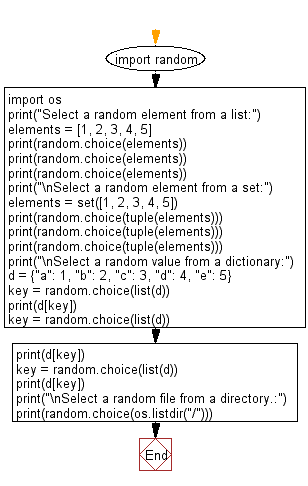﻿ Python: Select a random element from a list, set, dictionary and a file from a directory - w3resource# Python: Select a random element from a list, set, dictionary and a file from a directory

## Python module: Exercise-2 with Solution

Write a Python program to select a random element from a list, set, dictionary (value) and a file from a directory.

Use random.choice

Sample Solution:

Python Code:

``````import random
import os
print("Select a random element from a list:")
elements = [1, 2, 3, 4, 5]
print(random.choice(elements))
print(random.choice(elements))
print(random.choice(elements))
print("\nSelect a random element from a set:")
elements = set([1, 2, 3, 4, 5])
# convert to tuple because sets are invalid inputs
print(random.choice(tuple(elements)))
print(random.choice(tuple(elements)))
print(random.choice(tuple(elements)))
print("\nSelect a random value from a dictionary:")
d = {"a": 1, "b": 2, "c": 3, "d": 4, "e": 5}
key = random.choice(list(d))
print(d[key])
key = random.choice(list(d))
print(d[key])
key = random.choice(list(d))
print(d[key])
print("\nSelect a random file from a directory.:")
print(random.choice(os.listdir("/")))
```
```

Sample Output:

```Select a random element from a list:
3
4
5

Select a random element from a set:
1
3
5

Select a random value from a dictionary:
5
4
4

Select a random file from a directory.:
proc
```

Flowchart:## Visualize Python code execution:

The following tool visualize what the computer is doing step-by-step as it executes the said program:

Python Code Editor:

Have another way to solve this solution? Contribute your code (and comments) through Disqus.

What is the difficulty level of this exercise?

Test your Python skills with w3resource's quiz

﻿

## Python: Tips of the Day

For-else construct useful when searched for something and find it:

```# For example assume that I need to search through a list and process each item until a flag item is found and
# then stop processing. If the flag item is missing then an exception needs to be raised.

for i in mylist:
if i == theflag:
break
process(i)
else:
raise ValueError("List argument missing terminal flag.")
```